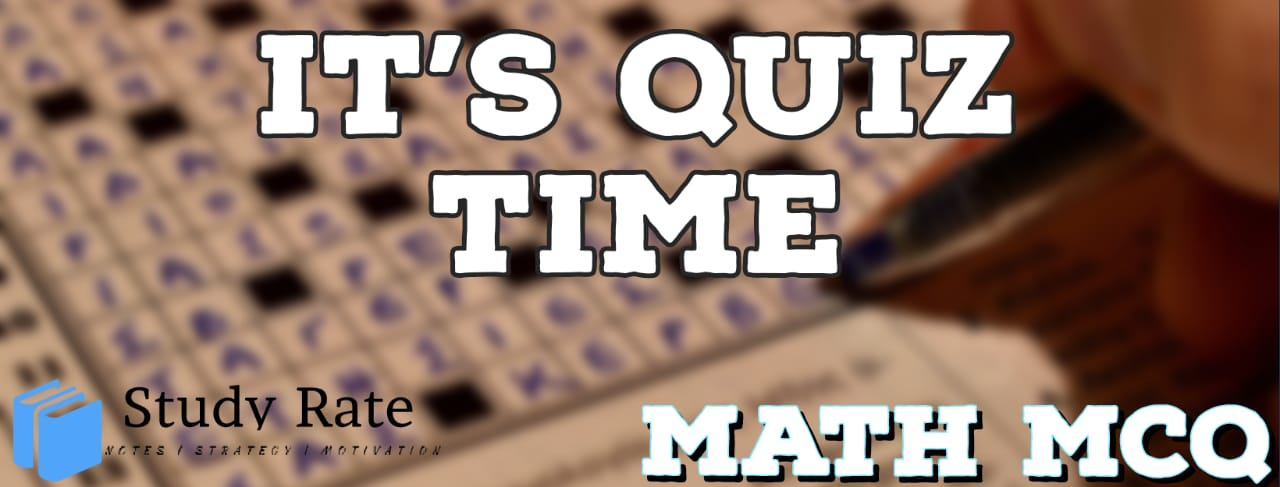## CBSE Class 10 Maths – Pair of Linear Equations in Two Variables MCQ Quiz

Free PDF Download of CBSE Class 10 Maths Chapter 3 Pair of Linear Equations in Two Variables Multiple Choice Questions with Answers. MCQ Questions for Class 10 Maths with Answers was Prepared Based on Latest Exam Pattern. Students can solve Class 10 Maths Pair of Linear Equations in Two Variables MCQs with Answers to know their preparation level

1. Half the perimeter of a rectangular room is 46 m, and its length is 6 m more than its breadth. What is the length and breadth of the room?

2. Solve3. Choose the pair of equations that satisfy the point (1,-1)4. The pair of equations x + 2y – 5 = 0 and −3x – 6y + 15 = 0 have:

5. If a pair of linear equations is consistent, then the lines will be:

6. The pair of equations y = 0 and y = –7 has

7.  If the lines given by
3x + 2ky = 2
2x + 5y + 1 = 0
are parallel, then the value of k is

8. The value of c for which the pair of equations cx – y = 2 and 6x – 2y = 3 will have infinitely many solutions are

9. One equation of a pair of dependent linear equations is –5x + 7y – 2 = 0. The second equation can be

10. The figure shows the graphical representation of a pair of linear equations. On the basis of graph, the pair of linear equation gives _______________solutions.11. Two numbers are in the ratio 5 : 6. If 8 is subtracted from each of the numbers, the ratio becomes 4 : 5. Then the numbers are:

12. The solution of the equations x – y = 2 and x + y = 4 is:

13. For which values of a and b, will the following pair of linear equations have infinitely many solutions?
x + 2y = 1
(a – b)x + (a + b)y = a + b – 2

14. The father’s age is six times his son’s age. Four years hence, the age of the father will be four times his son’s age. The present ages, in years, of the son and the father, are, respectively

15. In a competitive examination, one mark is awarded for each correct answer while 1/2 mark is deducted for every wrong answer. Jayanti answered 120 questions and got 90 marks.
How many questions did she answer correctly?